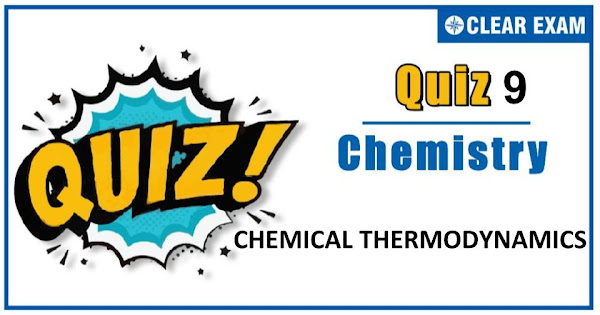## CHEMICAL THERMODYNAMICS QUIZ-9

The one subject in NEET which is candidates who can easily attain good marks is Chemistry. That's the reason, often, one doesn’t pay notice and choose to compromise it. But if one wants to rank above others, the tip is to be thorough with NEET chemistry concepts. The understanding of reactions and definite basic understanding is what requires major attention in Chemistry but once done it only gets simpler from there. The main focus on the to-do list should be on getting a hang of the NCERT syllabus of NEET chemistry..

Q1. Given that ∆H(r298 K)=-54.07 kJ mol-1 and ∆S(r298 K)°=10 J mol-1 and R=8.314 JK-1 mol-1. The value of log10⁡K for a reaction, A⇌B is:
•  5
•  10
•  95
•  100
Solution
b) ∆G°=∆H°-T∆S°
=-54.07-298×10×10-3
=-57.05 kJ
Also, ∆G°=2.303 RT log_10⁡K
log10⁡K=(-57.05×103)/(2.303×8.314×298)
Q2.A thermodynamic state function is:
•  One which obeys all the laws of thermodynamics
•  A quantity which is used to measure thermal changes
•  A quantity whose value is independent of the path
•  A quantity which is used to express pressure-volume work
Solution
c) The fact for a quantity referred as state function
Q3.  The work done by a system in an expansion against a constant external pressure is:
•  ∆P∙∆V
•  -P∙∆V
•  Q
•  V∙∆P
Solution
(b) Wirr. For a process at constant pressure =-P∙∆V; note that work is irreversible if expansion is made at constant pressure.

Q4. ∆H for the reaction given below represents,
CO2(g)+H2(g)⟶CO(g)+H2O(g); ∆H=40 kJ:
•  Heat of formation
•  Heat of combustion
•  Heat of neutralisation
•  Heat of reaction
Solution
(d) Heat changes during any chemical reaction are referred as heat of reaction are referred as heat of reaction for that change.
Q5.∆So will be highest for the reaction
•  Ca(s)+1/2 O2(g)→CaO(s)
•  CaCO3(g)→CaO(s)+CO2(g)
•  C(g)+O2(g)→CO2(g)
•  N2(g)+O2(g)→2NO(g)
Solution
b) Eq.(b) shows largest phase change ie,gas → solid
Q6. The enthalpy of dissolution of BaCl2(s) and BaCl2∙2H2O(s) are -20.6 and 8.8 kJ per mol
respectively.The enthalpy of hydration for, BaCl2(s)+2H2O→BaCl2∙2H2O(s) is
•  29.4 kJ
•  -29.4 kJ
•  -11.8 kJ
•  38.2 kJ
Solution
b)
Q7.A thermodynamic quantity is that:
•  Which is used in thermochemistry
•  Which obeys all the laws of thermodynamics
•  Quantity which depends only on the state of the system
•  Quantity which is used in measuring thermal change
Solution
c) e.g.,U,H,S, etc.
Q8.The heat evolved during the combustion of 112 litre of water gas (mixture of equal volume of H2 and CO) is :
H2(g)+1/2 O2(g)→H2O(g); ∆H= -241.8 kJ

CO(g)+1/2 O2(g)→CO2(g); ∆H= -283 kJ
•   241.8 kJ
•  283 kJ
•  -1312 kJ
•  1586 kJ
Solution
(c) ∆H for combustion of 56 litre H2= (- 24.1 × 56)/22.4
∆H for combustion of 56 litre CO=(-263 × 56)/22.4
∴ Total ∆H= -1312 kJ
Q9. The enthalpy of formation of HI is 30.4 kJ. Which statement is false according to this observation?
•  HI is an endothermic compound
•  For the reaction,H2(g)+I2(g)⟶2HI(g);∆H=60.8 kJ
•  HI is a stable compound
•  HI is an unstable compound
Solution
(c) Due to positive ∆H, HI is endothermic compound and unstable.
Q10. Select the incorrect statement
•  PV work is usually negligible for solid and liquid
•  For a closed system with P-V work only, an isobaric process that has q=+ve must have ∆T=+ve.
•  For a cyclic process q=0
•  Black phosphorus is most stable form of P but H°f=0 for white phosphorus.
Solution
(b) Rest all are correct .#### Written by: AUTHORNAME

AUTHORDESCRIPTION## Want to know more

Please fill in the details below:

## Latest NEET Articles\$type=three\$c=3\$author=hide\$comment=hide\$rm=hide\$date=hide\$snippet=hide

Name

ltr
item
BEST NEET COACHING CENTER | BEST IIT JEE COACHING INSTITUTE | BEST NEET, IIT JEE COACHING INSTITUTE: Chemical Thermodynamics Quiz-9
Chemical Thermodynamics Quiz-9
https://1.bp.blogspot.com/-b6YqiKCWugs/X6HBy0sF2wI/AAAAAAAAJk8/tNItv7K4YeYUYn57nucuo0aBS8UA0BMnQCLcBGAsYHQ/s600/Quiz%2BImage%2B20%2B%25288%2529.jpg
https://1.bp.blogspot.com/-b6YqiKCWugs/X6HBy0sF2wI/AAAAAAAAJk8/tNItv7K4YeYUYn57nucuo0aBS8UA0BMnQCLcBGAsYHQ/s72-c/Quiz%2BImage%2B20%2B%25288%2529.jpg
BEST NEET COACHING CENTER | BEST IIT JEE COACHING INSTITUTE | BEST NEET, IIT JEE COACHING INSTITUTE
https://www.cleariitmedical.com/2020/11/chemical-thermodynamics-quiz-9.html
https://www.cleariitmedical.com/
https://www.cleariitmedical.com/
https://www.cleariitmedical.com/2020/11/chemical-thermodynamics-quiz-9.html
true
7783647550433378923
UTF-8